# What is Electricity ?

#### What is electricity?

Electricity is defined as “the flow of electrons through simple materials and devices” or “that force which moves electrons.” Scientists think electricity is produced by very tiny particles called electrons and protons. These particles are too small to be seen, but exist as subatomic particles in the atom.

To understand how they exist, you must first understand the structure of the atom.

#### The Atom

Elements are the basic building blocks of all matter. The atom is the smallest particle to which an element can be reduced while still keeping the properties of that element. An atom consists of a positively charged nucleus surrounded by negatively charged electrons, so that the atom as a whole is electrically neutral. The nucleus is composed of two kinds of subatomic particles, protons and neutrons, as shown in Figure 1.

The proton carries a single unit positive charge equal in magnitude to the electron charge. The neutron is slighty heavier than the proton and is electrically neutral, as the name implies. These two particles exist in various combinations, depending upon the element involved. The electron is the fundamental negative charge (-) of electricity and revolves around the nucleus, or center, of the atom in concentric orbits, or shells.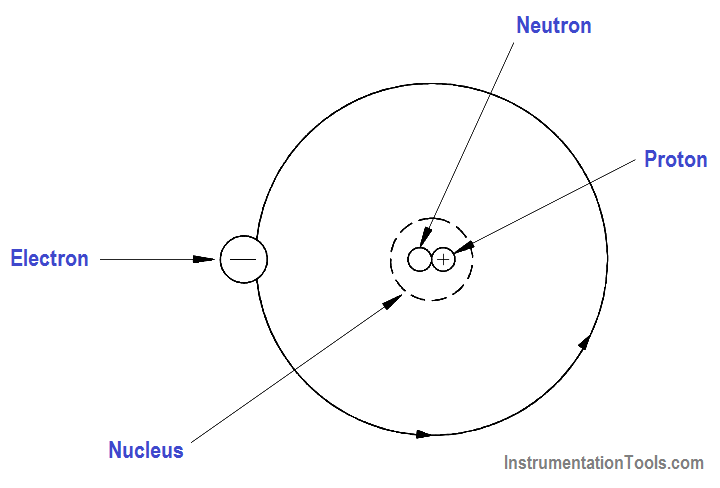Figure 1 – The Atom

The proton is the fundamental positive charge (+) of electricity and is located in the nucleus. The number of protons in the nucleus of any atom specifies the atomic number of that atom or of that element. For example, the carbon atom contains six protons in its nucleus; therefore, the atomic number for carbon is six, as shown in Figure 2.

In its natural state, an atom of any element contains an equal number of electrons and protons. The negative charge (-) of each electron is equal in magnitude to the positive charge (+) of each proton; therefore, the two opposite charges cancel, and the atom is said to be electrically neutral, or in balance.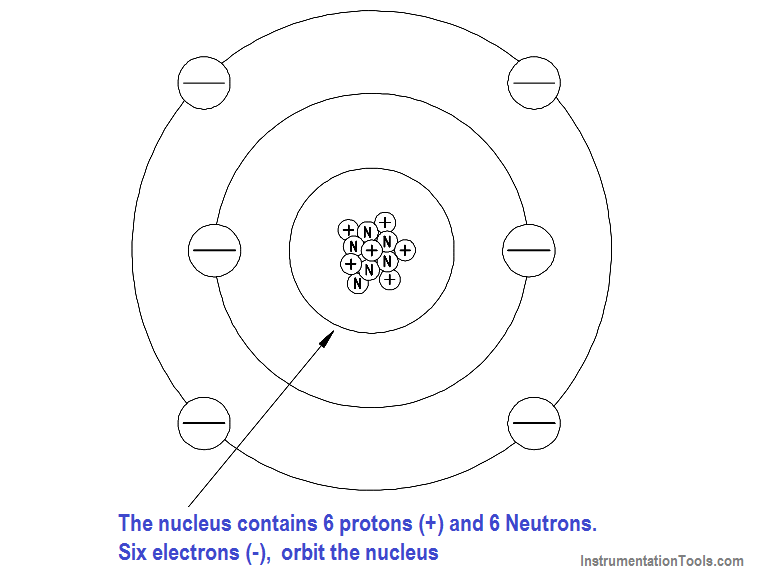Figure 2 – The Carbon Atom

#### Electrostatic Force

One of the mysteries of the atom is that the electron and the nucleus attract each other. This attraction is called electrostatic force, the force that holds the electron in orbit. This force may be illustrated with lines as shown in Figure 3.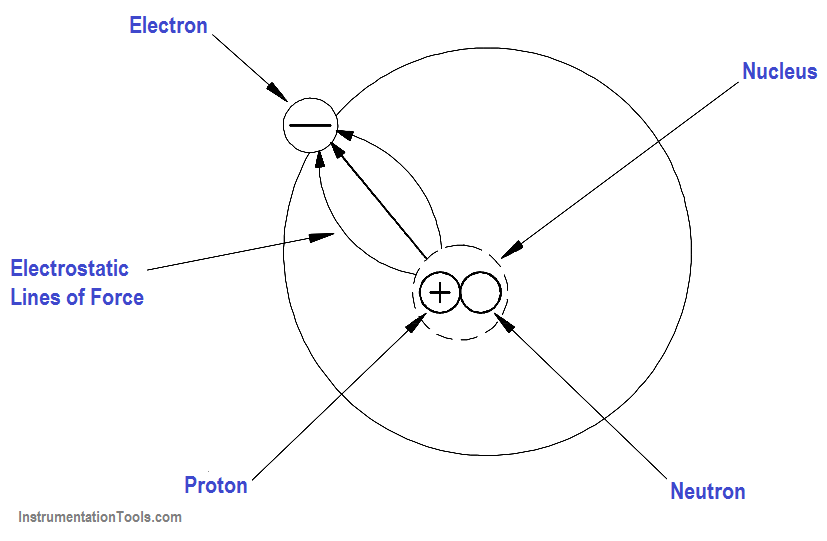Figure 3 – Electrostatic Force

Without this electrostatic force, the electron, which is traveling at high speed, could not stay in its orbit. Bodies that attract each other in this way are called charged bodies. As mentioned previously, the electron has a negative charge, and the nucleus (due to the proton) has a positive charge.

#### The First Law of Electrostatics

The negative charge of the electron is equal, but opposite to, the positive charge of the proton. These charges are referred to as electrostatic charges. In nature, unlike charges (like electrons and protons) attract each other, and like charges repel each other. These facts are known as the First Law of Electrostatics and are sometimes referred to as the law of electrical charges. This law should be remembered because it is one of the vital concepts in electricity.

Some atoms can lose electrons and others can gain electrons; thus, it is possible to transfer electrons from one object to another. When this occurs, the equal distribution of negative and positive charges no longer exists. One object will contain an excess of electrons and become negatively charged, and the other will become deficient in electrons and become positively charged. These objects, which can contain billions of atoms, will then follow the same law of electrostatics as the electron and proton example shown above.

The electrons that can move around within an object are said to be free electrons and will be discussed in more detail in a later section. The greater the number of these free electrons an object contains, the greater its negative electric charge. Thus, the electric charge can be used as a measure of electrons.

#### Electrostatic Field

A special force is acting between the charged objects discussed above. Forces of this type are the result of an electrostatic field that exists around each charged particle or object. This electrostatic field, and the force it creates, can be illustrated with lines called “lines of force” as shown in Figure 4.Figure 4 – Electrostatic Field

Charged objects repel or attract each other because of the way these fields act together. This force is present with every charged object. When two objects of opposite charge are brought near one another, the electrostatic field is concentrated in the area between them, as shown in Figure 5. The direction of the small arrows shows the direction of the force as it would act upon an electron if it were released into the electric field.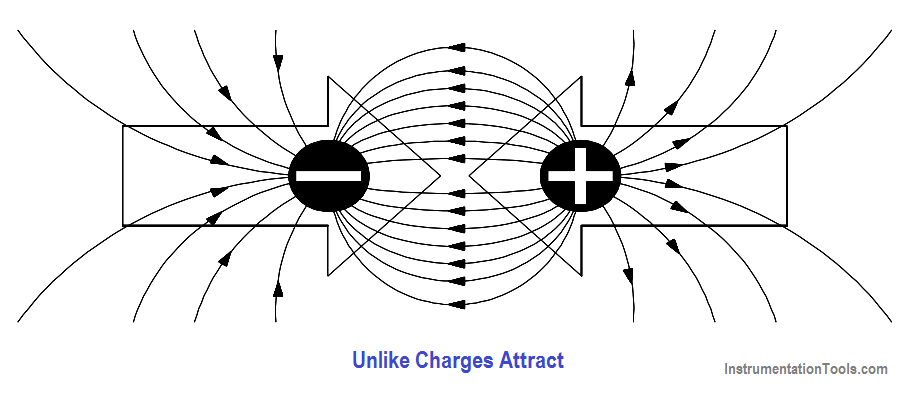Figure 5 – Electrostatic Field Between Two Charges of Opposite Polarity

When two objects of like charge are brought near one another, the lines of force repel each other, as shown in Figure 6.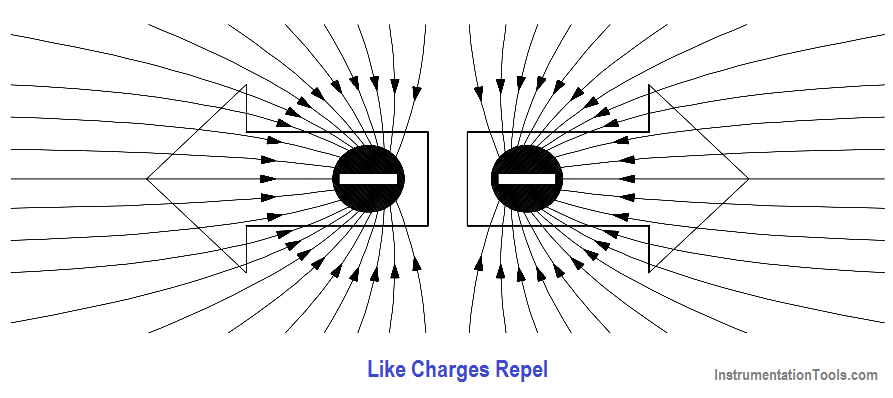Figure 6 – Electrostatic Field Between Two Charges of Like Polarity

The strength of the attraction or of the repulsion force depends upon two factors:

1. the amount of charge on each object, and
2. the distance between the objects.

The greater the charge on the objects, the greater the electrostatic field. The greater the distance between the objects, the weaker the electrostatic field between them, and vice versa. This leads us to the law of electrostatic attraction, commonly referred to as Coulomb’s Law of electrostatic charges, which states that the force of electrostatic attraction, or repulsion, is directly proportional to the product of the two charges and inversely proportional to the square of the distance between them as shown in Equation 1-1.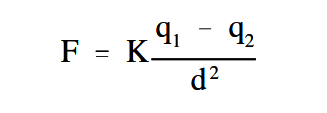where

F = force of electrostatic attraction or prepulsion (Newtons)
K = constant of proportionality (Coulomb2 /N-m2 )
q1 = charge of first particle (Coulombs)
q2 = charge of second particle (Coulombs)
d = distance between two particles (Meters)

If q1 and q2 are both either positively or negatively charged, the force is repulsive. If q1 and q2 are opposite polarity or charge, the force is attractive.

#### Potential Difference

Potential difference is the term used to describe how large the electrostatic force is between two charged objects. If a charged body is placed between two objects with a potential difference, the charged body will try to move in one direction, depending upon the polarity of the object. If an electron is placed between a negatively-charged body and a positively-charged body, the action due to the potential difference is to push the electron toward the positively-charged object. The electron, being negatively charged, will be repelled from the negatively-charged object and attracted by the positively-charged object, as shown in Figure 7.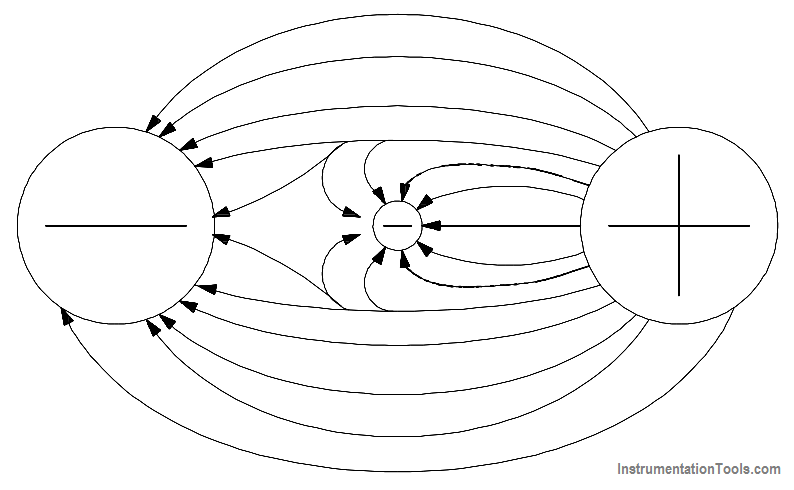Figure 7 – Potential Difference Between Two Charged Objects

Due to the force of its electrostatic field, these electrical charges have the ability to do work by moving another charged particle by attraction and/or repulsion. This ability to do work is called “potential”; therefore, if one charge is different from another, there is a potential difference between them. The sum of the potential differences of all charged particles in the electrostatic field is referred to as electromotive force (EMF).

The basic unit of measure of potential difference is the “volt.” The symbol for potential difference is “V,” indicating the ability to do the work of forcing electrons to move. Because the volt unit is used, potential difference is also called “voltage.”

#### Free Electrons

Electrons are in rapid motion around the nucleus. While the electrostatic force is trying to pull the nucleus and the electron together, the electron is in motion and trying to pull away. These two effects balance, keeping the electron in orbit. The electrons in an atom exist in different energy levels. The energy level of an electron is proportional to its distance from the nucleus. Higher energy level electrons exist in orbits, or shells, that are farther away from the nucleus. These shells nest inside one another and surround the nucleus.

The nucleus is the center of all the shells. The shells are lettered beginning with the shell nearest the nucleus: K, L, M, N, O, P, and Q. Each shell has a maximum number of electrons it can hold. For example, the K shell will hold a maximum of two electrons and the L shell will hold a maximum of eight electrons. As shown in Figure 8, each shell has a specific number of electrons that it will hold for a particular atom.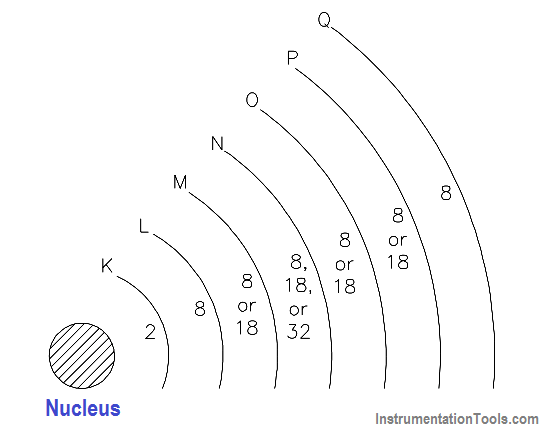Figure 8- Energy Shells and Electron Quota

There are two simple rules concerning electron shells that make it possible to predict the electron distribution of any element:

1. The maximum number of electrons that can fit in the outermost shell of any atom is eight.
2. The maximum number of electrons that can fit in the next-to-outermost shell of any atom is 18.

An important point to remember is that when the outer shell of an atom contains eight electrons, the atom becomes very stable, or very resistant to changes in its structure. This also means that atoms with one or two electrons in their outer shell can lose electrons much more easily than atoms with full outer shells.

The electrons in the outermost shell are called valence electrons. When external energy, such as heat, light, or electrical energy, is applied to certain materials, the electrons gain energy, become excited, and may move to a higher energy level. If enough energy is applied to the atom, some of the valence electrons will leave the atom. These electrons are called free electrons. It is the movement of free electrons that provides electric current in a metal conductor. An atom that has lost or gained one or more electrons is said to be ionized or to have an ion change. If the atom loses one or more electrons, it becomes positively charged and is referred to as a positive ion. If an atom gains one or more electrons, it becomes negatively charged and is referred to as a negative ion.

Summary